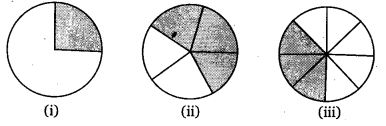# Class 7 Maths NCERT Solutions for Chapter – 8 Comparing Quantities Ex – 8.2

## Comparing Quantities

Question 1.
Convert the given fractional number to per cents :
(a)$\frac { 1 }{ 8 }$
(b)$\frac { 5 }{ 4 }$
(c)$\frac { 3 }{ 40 }$
(d)$\frac { 2 }{ 7 }$

Solution:

Question 2.
Convert the given decimal fractions to per cents.
(a)
0.65
(b) 2.1
(c) 0.02
(d) 12.35

Solution:

Question 3.
Estimate what part of the figures is coloured and hence find the per cent which is coloured.Solution:

Question 4.
Find :
(a)
15% of 250
(b) 1% of 1 hour
(c) 20% of ₹ 2500
(d) 75% of 1 kg

Solution:

Question 5.
Find the whole quantity if
(a)
5% of it is 600
(b) 12% of it is ? 1080
(c) 40% of it is 500 km
(d) 70% of it is 14 minutes
(e) 8% of it is 40 litres

Solution:

Question 6.
Convert given per cents to decimal fractions and also to fractions in simplest forms :
(a)
25%
(b) 150%
(c) 20%
(d) 5%

Solution:

Question 7.
In a city, 30% are females, 40% are males and remaining are children. What per cent are children?

Solution:
In a city,
Percentage of females = 30%
Percentage of males = 40%
Percentage of children = (100 – 30 – 40)% = 30%

Question 8.
Out of 15,000 voters in a constituency, 60% voted. Find the percentage of voters who did not vote. Can you now find how many actually did not vote?

Solution:
Percentage of voters who voted = 60%
Percentage of voters who did not vote = (100 – 60)% = 40%
Total number of voters = 15000
Number of voters who did not vote
= 40% of 15000
= ($\frac { 40 }{ 100 }$ × 15000 ) = 6000

Question 9.
Meeta saves ₹ 400 from her salary. If this is 10% of her salary, what is her salary?

Solution:

Question 10.
A local cricket team played 20 matches in one season. It won 25% of them. How many matches did they win?

Solution:
Out of 100, 25% matches are won. Then, out of 20, the number of matches that the team won$\frac { 25 }{ 100 }$ × 20 =$\frac { 1 }{ 4 }$ × 20 = 5